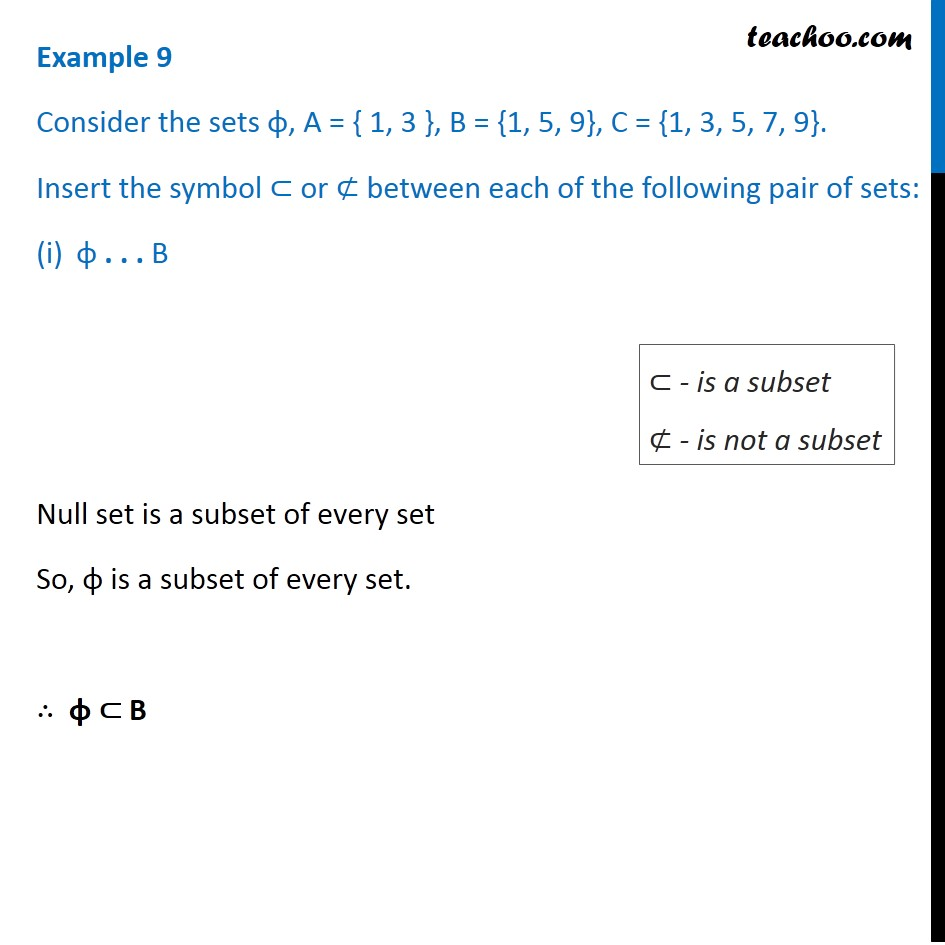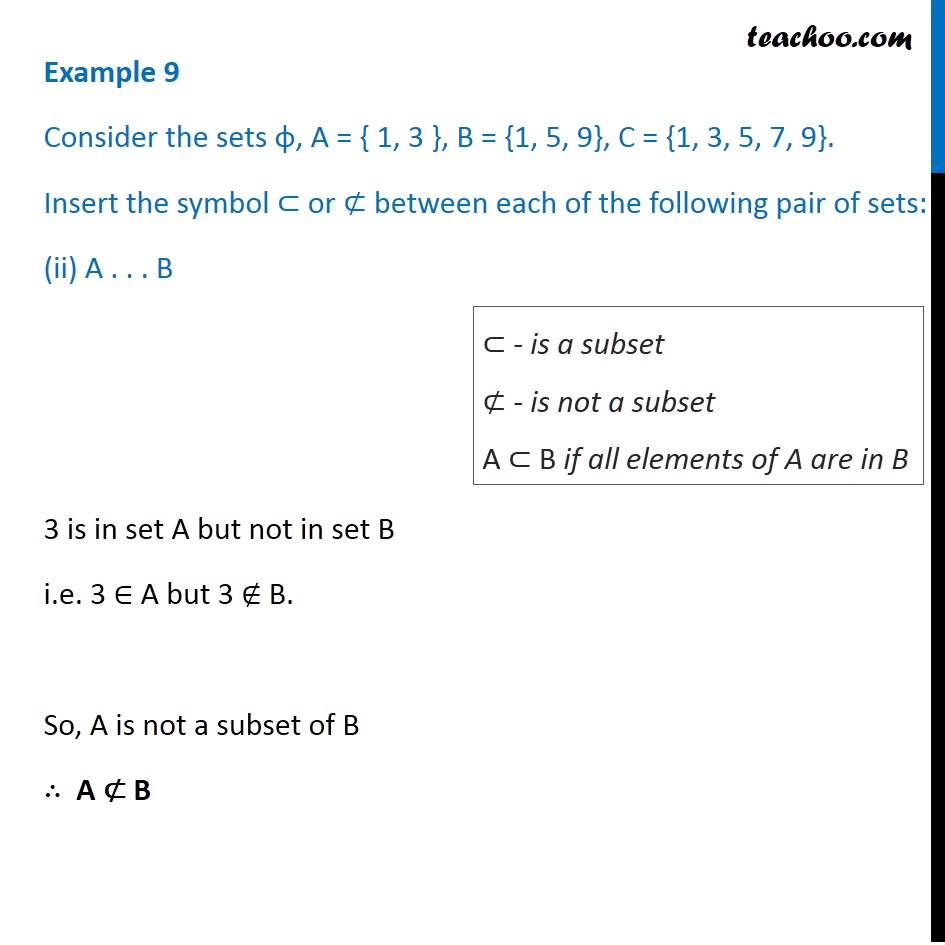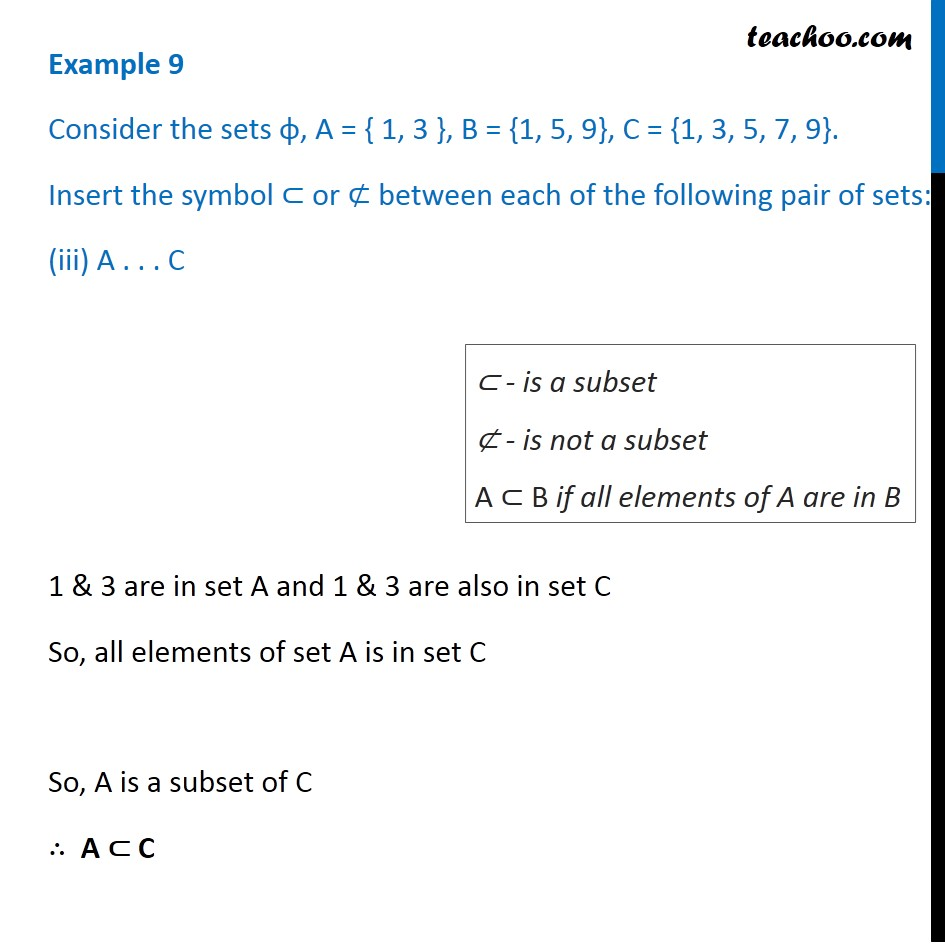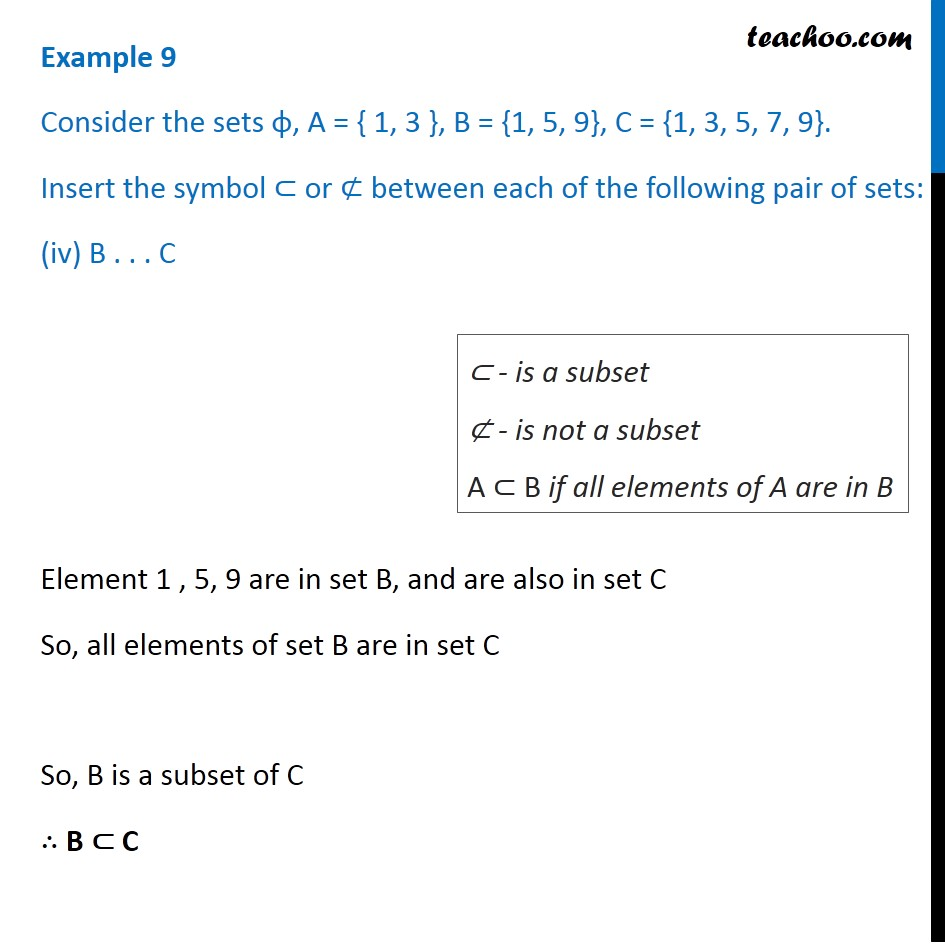Learn about Sets on our Youtube Channel - https://you.tube/Chapter-1-Class-11-Sets1. Chapter 1 Class 11 Sets
2. Concept wise
3. Subset

Transcript

Example 9 Consider the sets φ, A = { 1, 3 }, B = {1, 5, 9}, C = {1, 3, 5, 7, 9}. Insert the symbol ⊂ or ⊄ between each of the following pair of sets: φ . . . B Null set is a subset of every set So, φ is a subset of every set. ∴ φ ⊂ B ⊂ - is a subset ⊄ - is not a subset Example 9 Consider the sets φ, A = { 1, 3 }, B = {1, 5, 9}, C = {1, 3, 5, 7, 9}. Insert the symbol ⊂ or ⊄ between each of the following pair of sets: (ii) A . . . B 3 is in set A but not in set B i.e. 3 ∈ A but 3 ∉ B. So, A is not a subset of B ∴ A ⊄ B ⊂ - is a subset ⊄ - is not a subset A ⊂ B if all elements of A are in B Example 9 Consider the sets φ, A = { 1, 3 }, B = {1, 5, 9}, C = {1, 3, 5, 7, 9}. Insert the symbol ⊂ or ⊄ between each of the following pair of sets: (iii) A . . . C 1 & 3 are in set A and 1 & 3 are also in set C So, all elements of set A is in set C So, A is a subset of C ∴ A ⊂ C ⊂ - is a subset ⊄ - is not a subset A ⊂ B if all elements of A are in B Example 9 Consider the sets φ, A = { 1, 3 }, B = {1, 5, 9}, C = {1, 3, 5, 7, 9}. Insert the symbol ⊂ or ⊄ between each of the following pair of sets: (iv) B . . . C Element 1 , 5, 9 are in set B, and are also in set C So, all elements of set B are in set C So, B is a subset of C ∴ B ⊂ C ⊂ - is a subset ⊄ - is not a subset A ⊂ B if all elements of A are in B

Subset

About the AuthorDavneet Singh
Davneet Singh is a graduate from Indian Institute of Technology, Kanpur. He has been teaching from the past 9 years. He provides courses for Maths and Science at Teachoo.